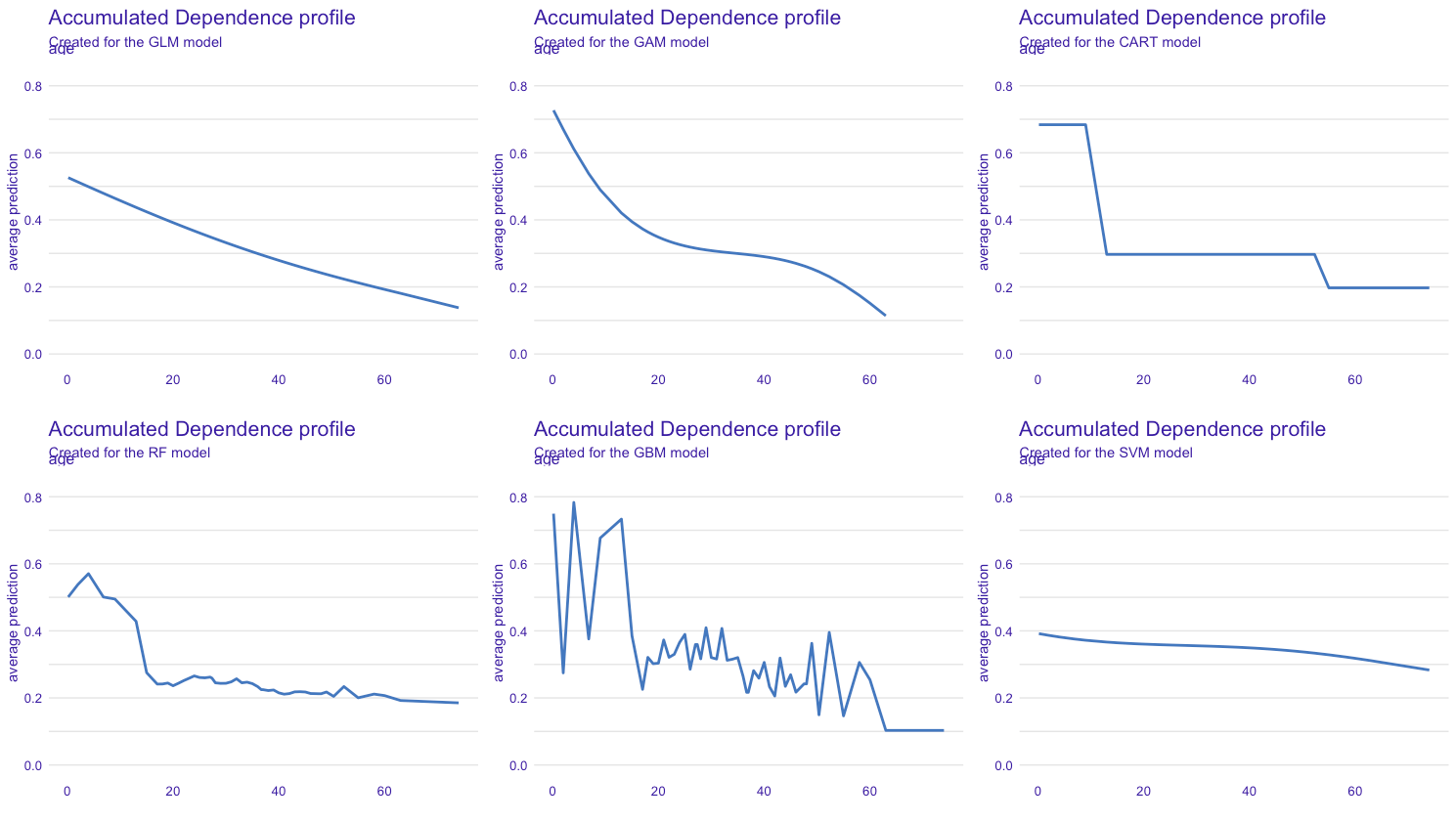Want to share your content on R-bloggers? click here if you have a blog, or here if you don't.

In 400 AD, in his Confessiones, Augustine wrote

quid est ergo tempus? si nemo ex me quaerat, scio; si quaerenti explicare velim, nescio

that can be translated as

What then is time? If no one asks me, I know what it is. If I wish to explain it to him who asks, I do not know.

To go a little further (because often, if we are asked to explain, we have some ideas), in A Study in Scarlet by Sir Arthur Conan Doyle, published in 1887, we have the following exchange, between Sherlock Holmes and Doctor Watson

– “I wonder what that fellow is looking for?” I asked, pointing to a stalwart, plainly-dressed individual who was walking slowly down the other side of the street, looking anxiously at the numbers. He had a large blue envelope in his hand, and was evidently the bearer of a message.
– “You mean the retired sergeant of Marines,” said Sherlock Holmes.

then, as it turns out that the person is indeed a sergeant in the navy (as is another character in the story, someone named Arthur Charpentier), Dr. Holmes asks him for an explanation, he wants to know how he arrived at this conclusion

– “How in the world did you deduce that?” I asked.
“Deduce what?” said he, petulantly.
“Why, that he was a retired sergeant of Marines.”
“I have no time for trifles,” he answered, brusquely; then with a smile, “Excuse my rudeness. You broke the thread of my thoughts; but perhaps it is as well. So you actually were not able to see that that man was a sergeant of Marines?”
“No, indeed.”
– “It was easier to know it than to explain why I knew it. If you were asked to prove that two and two made four, you might find some difficulty, and yet you are quite sure of the fact. Even across the street I could see a great blue anchor tattooed on the back of the fellow’s hand. That smacked of the sea. He had a military carriage, however, and regulation side whiskers. There we have the marine. He was a man with some amount of self-importance and a certain air of command. You must have observed the way in which he held his head and swung his cane. A steady, respectable, middle-aged man, too, on the face of him – all facts which led me to believe that he had been a sergeant.”

(to be honest, it is Liu Cixin who talks about it in The Three-Body Problem). For the record, this is the first story of the Holmes-Watson couple, which introduces Sherlock Holmes’ working method. For those who are familiar with the short stories, this narrative approach will be widely used thereafter: Sherlock Holmes states a fact, Dr. Watson is astonished and asks for an explanation, and Sherlock Holmes explains, point by point, how he arrived at this conclusion. This is a bit like the approach we try to implement when we build a predictive model: on the basis of the Titanic data, if we predict that such and such a person will die, and that such and such a person will survive, we want to understand why the model arrives at this conclusion.

Beyond the general reflections, from “linear models are not as simple to interpret and explain as they seem” to “one can explain without being able to predict, and predict without being able to explain”, I wanted to come back to the classical mathematical notions used when we talk about the explainability of predictive models. On the basis of the Titanic data,

library(DALEX)
data("titanic")
titanic = DALEX::titanic
idx = which((is.na(titanic$age))+ (is.na(titanic$sibsp))+
(is.na(titanic$parch))==0) titanicNA = titanic[idx,] we will consider six different models to predict survivorship • a logistic GLM and a GAM version (with a spline on the age) • a classification tree CART and a random forest RF • a boosting model GBM and a support vector machine SVM library(splines) titanic_glm = glm(survived == "yes" ~ gender + age + class + sibsp + parch + embarked, titanicNA, family="binomial") titanic_gam = glm(survived == "yes" ~ gender + bs(age) + class + sibsp + parch + embarked, titanicNA, family="binomial") library("rpart") titanic_cart = rpart(survived == "yes" ~ class + gender + age + sibsp + parch + embarked, data = titanicNA) library("gbm") set.seed(1234) titanic_gbm = gbm(survived == "yes" ~ class + gender + age + sibsp + parch + embarked, data = titanicNA, n.trees = 15000, distribution = "bernoulli") library("randomForest") set.seed(1234) titanic_rf = randomForest(survived ~ class + gender + age + sibsp + parch + embarked, data = titanicNA) library("e1071") titanic_svm = svm(survived == "yes" ~ class + gender + age + sibsp + parch + embarked, data = titanicNA, type = "C-classification", probability = TRUE) and to try to explain, we will see local methods, with two fictitious passengers, Kate and Leonardo (as I had already done in the past). newbase = data.frame( class = factor(c("1st","3rd"), levels = c("1st", "2nd", "3rd", "deck crew", "engineering crew", "restaurant staff", "victualling crew")), gender = factor(c("female","male"), levels = c("female", "male")), age = c(17,20), sibsp = c(1,0), parch = c(2,0), embarked = factor(c("Southampton","Southampton"), levels = c("Belfast","Cherbourg","Queenstown","Southampton"))) rownames(newbase) = c("Winslet, Miss. Kate","DiCaprio, Mr. Leonardo") We will use here, to illustrate, the DALEX R package, by Przemyslaw Biecek, detailed in the book writen with Tomasz Burzykowski, Explanatory model analysis, titanic_cart_exp = DALEX::explain(model = titanic_cart, data = titanicNA[, -9], y = titanicNA$survived == "yes",
label = "cart",
type = "classification")
titanic_glm_exp = DALEX::explain(model = titanic_glm,
data = titanicNA[, -9],
y = titanicNA$survived == "yes", label = "glm", type = "classification") titanic_gam_= DALEX::explain(model = titanic_gam, data = titanicNA[, -9], y = titanicNA$survived == "yes",
label = "gam",
type = "classification")
titanic_rf_exp = DALEX::explain(model = titanic_rf,
data = titanicNA[, -9],
y = titanicNA$survived == "yes", label = "rf") titanic_gbm_exp = DALEX::explain(model = titanic_gbm, data = titanicNA[, -9], y = titanicNA$survived == "yes",
label = "gbm")
titanic_svm_exp = DALEX::explain(model = titanic_svm,
data = titanicNA[, -9],
y = titanicNA\$survived == "yes",
label = "svm")

But first, let us return to the distinction between ceteris paribus and mutatis mutandis. Ceteris paribus (or rather ceteris paribus sic stantibus) is the Latin phrase that translates by “all things being equal”.  Mutatis mutandis translates as “what should be changed has been changed” or “once the necessary changes have been made”. This distinction will be important later on. To illustrate, consider a simple model $$\text{obesity}=m(\text{weight},\text{height})$$. In the first case, to understand the impact of the weight on obesity, we will consider $$m(\text{weight}=x+dx,\text{taille}=y)-m(\text{weight}=x,\text{taille}=y)$$ as on the picture below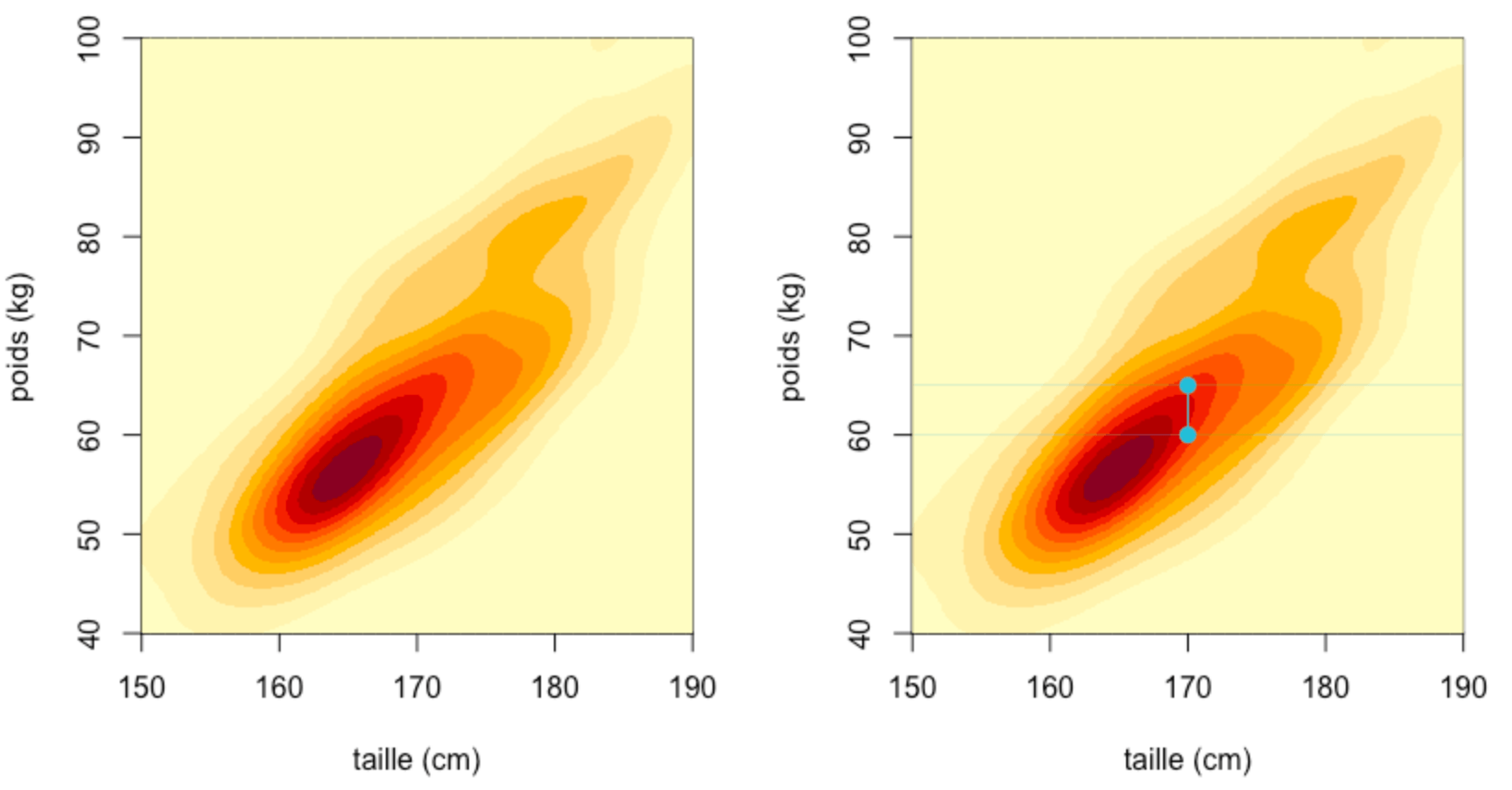In the second case, we want to take into account the fact that an individual of different weight would probably also be of different height, and so we should look at$$m(\text{weight}=x+dx,\text{taille}=y+\epsilon)-m(\text{weight}=x,\text{taille}=y)$$as on the picture below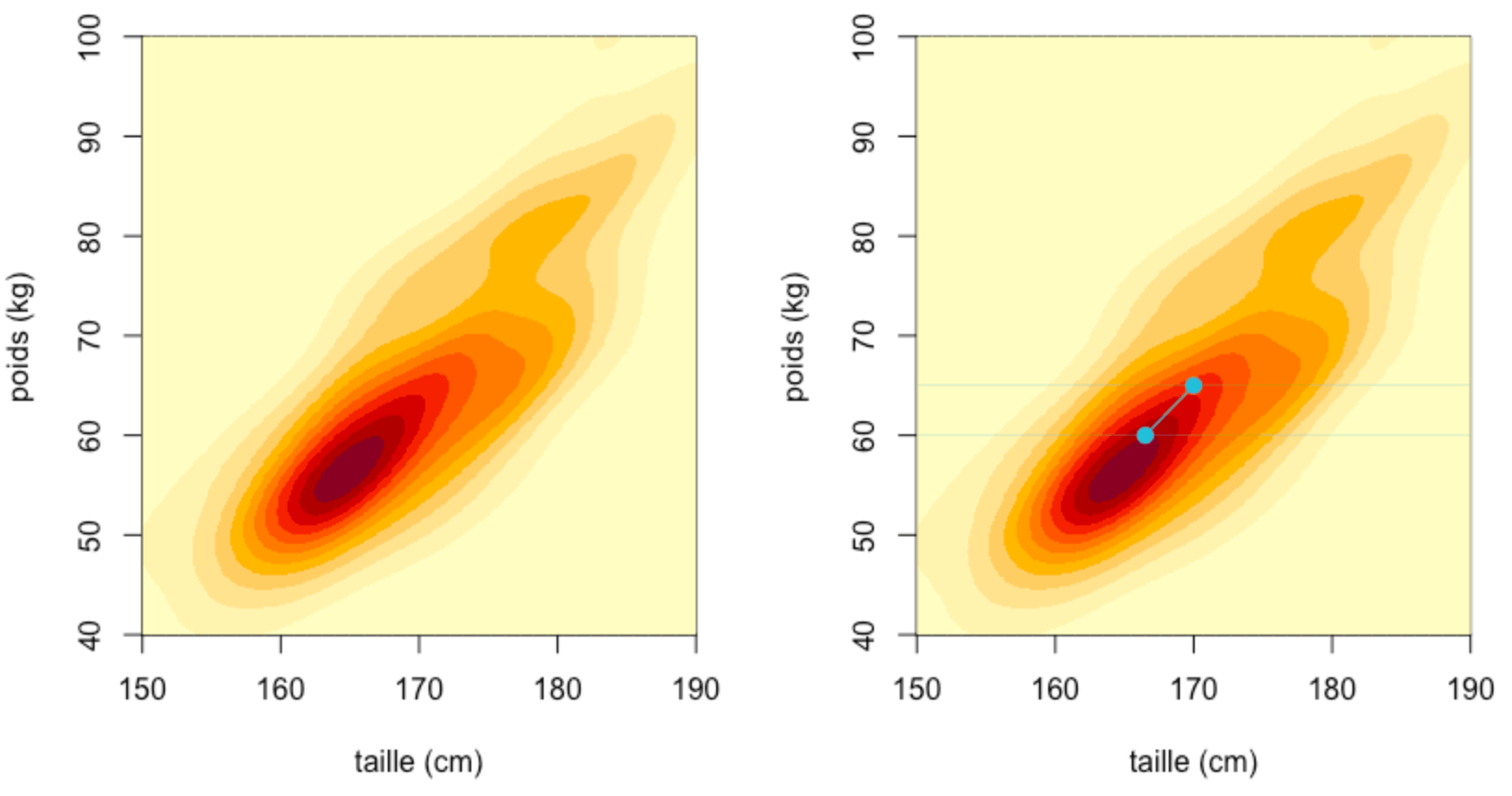where $$\epsilon$$ would integrate, somehow, the correlation between the two component.

To formalize this, consider a Gaussian model, that is two random variables $$(X_1,X_2)$$ (that might be the height and the weight, for instance) such that $$\begin{pmatrix}X_1\\X_2\end{pmatrix}\sim\mathcal{N}\left(\begin{pmatrix}\mu_1\\\mu_2\end{pmatrix},\begin{pmatrix}\sigma_1^2 & r\sigma_1\sigma_2 \\r\sigma_1\sigma_2 & \sigma_2^2\end{pmatrix}\right)$$. In that case, consider $$(X_1^\perp,X_2^\perp)$$ an independent version of $$(X_1,X_2)$$ (i.e. same marginal distributions, but independent). The (classical) conditional expectation can be written $$\displaystyle{\mathbb{E}_{X_1}[X_2|x_1^*]=\mu_2+\frac{r\sigma_2}{\sigma_1}(x_1^*-\mu_1)}$$and we will use the notation$$\displaystyle{\mathbb{E}_{X_1^\perp}[X_2|x_1^*]=\mathbb{E}[X_2]=\mu_2}$$in the case where components are independent.

This notation with the conditional random variable appearing as an index might be surprising, but here, $$\mathbb{E}_{X_1}$$ means that the expected value is not under $$\mathbb{P}$$, but under$$\mathbb{P}_{X_2|X_1}$$, also denoted, sometimes $$\mathbb{E}^{\mathbb{P}_{X_2|X_1}}$$. I will use it for convenience here. From a statistical perspective, the later one (with independent variables), it means that $$\mathbb{E}_{X_1^\perp}[h(X_1,X_2)|x_1^*] \approx \frac{1}{n}\sum_{i=1}^n h(x_{1}^*,x_{i,2})$$ while the true classical conditional expectation should be understood as the average in the neighborhood of $$x_1^*$$ and therefore $$\mathbb{E}_{X_1}[h(X_1,X_2)|x_1^*] \approx \frac{1}{\|\mathcal{V}_\epsilon(x_1^*)\|}\sum_{i\in\mathcal{V}_\epsilon(x_1^*)} h(x_{1}^*,x_{i,2})$$where $$\mathcal{V}_\epsilon(x_1^*)$$ precisely denotes the neighborhood, i.e.$$\mathcal{V}_\epsilon(x_1^*)=\big\lbrace i:|x_{i,1}-x_1^*|\leq \epsilon\big\rbrace$$

Before going forward, I want to stress here that we will talk about “local” explanability when we try to explain a single prediction, given by model $$m$$ for some very specific individual (denoted $$\boldsymbol{x}^*$$) while the “global” approach usually means that we want to quantify variable importance in model $$m$$ (and possibly, consider functions of $$x_j^*$$).

• Global Approach: variable importance

Variable importance is a first tool to understand which variables are important in a predictive model$$m$$. Fisher et al. (2019) suggested the following definition : given a loss function $$\ell$$, define$$VI_{j}=\mathbb{E}\big[\ell(Y,m(\boldsymbol{X}_{-j},X_j))\big]-\mathbb{E}\big[\ell(Y,m(\boldsymbol{X}_{-j},X_j^\perp))\big]$$and the empirical version is$$\widehat{VI}_{j}=\frac{1}{n}\sum_{i=1}^n \ell(y_i,m(\boldsymbol{x}_{i,-j},x_{i,j}))-\ell(y_i,m(\boldsymbol{x}_{i,-j},\tilde{x}_{i,j}))$$for some permutation $$\tilde{\boldsymbol{x}}_j$$ of vector $${\boldsymbol{x}}_j$$.

vip_glm_999 = model_parts(explainer = titanic_glm_exp,
loss_function = 1-AUC,
B = 999,
type = "difference"))
plot(vip_glm_999)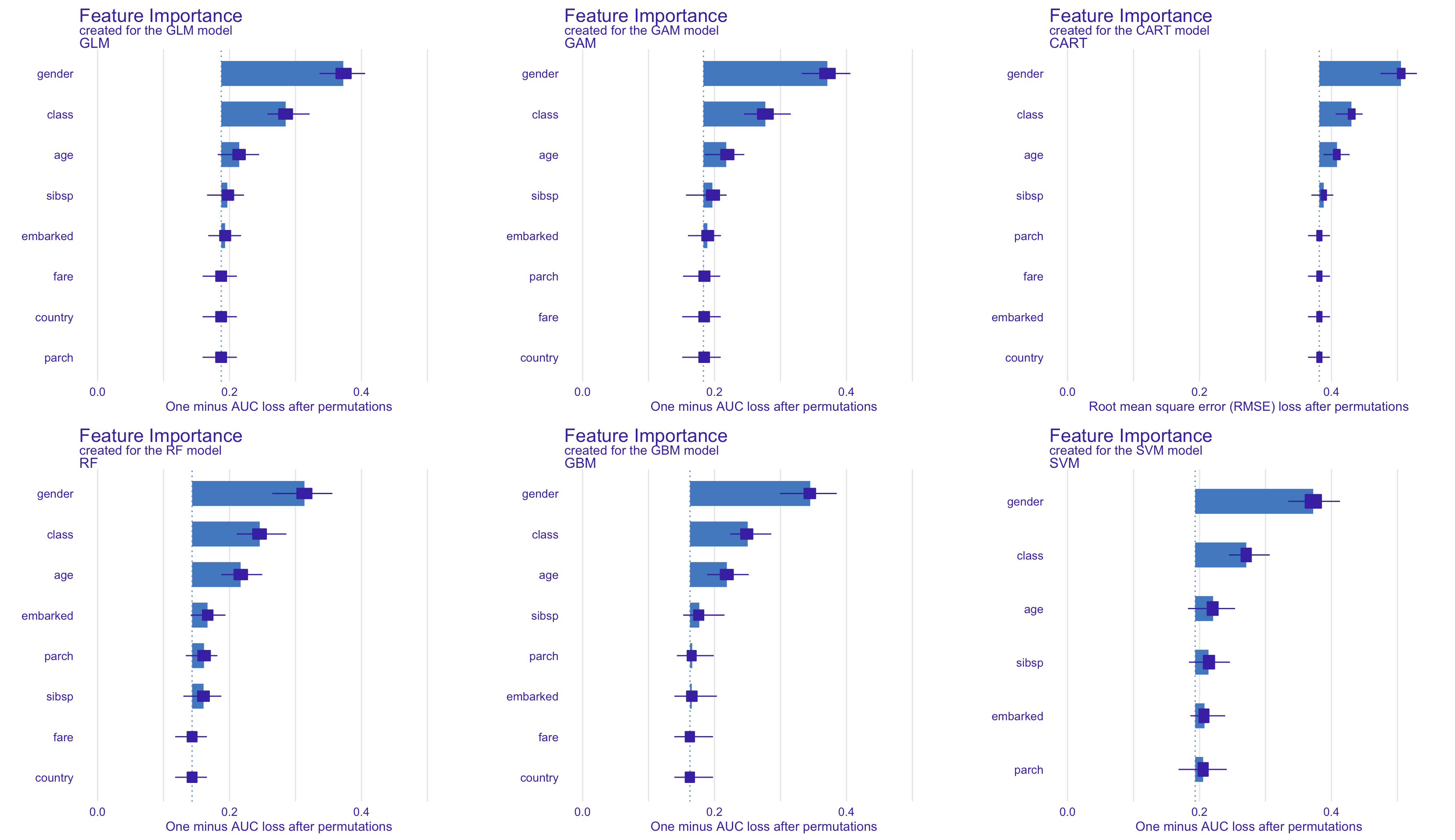• Local Approach : ICE or ceteris paribus

Goldstein et al. (2015) introduced the concept of ICE (individual conditional expectation), which is simply the functional ceteris paribus$$z\mapsto m_{\boldsymbol{x}^*,j}(z) = m(\boldsymbol{x}^*_{-j},z)=m(x_1^*,\cdots,x_{j-1}^*,z,x_{j+1}^*,\cdots,x_p^*)$$ in a given point $$\boldsymbol{x}^*\in\mathcal{X}$$  (with a small abuse of notation on the indices, since one will note abusively $$(\boldsymbol{x}^*_{-j},x_j^*)$$ whatever position of index $$j$$).

We can then look at $$\delta m_{\boldsymbol{x}^*,j}(z)=m_{\boldsymbol{x}^*,j}(z) – m_{\boldsymbol{x}^*,j}(x_j^*)$$, or more interestingly, The mean absolute deviation of the $$j$$-th variable, in $$\boldsymbol{x}^*$$, is $$dm_{j}(\boldsymbol{x}^*)$$ $$\displaystyle{dm_{j}(\boldsymbol{x}^*) =\mathbb{E}\big[|\delta m_{\boldsymbol{x}^*, j}(X_j)|\big] =\mathbb{E}\big[|m(\boldsymbol{x}_{-j},X_j) – m(\boldsymbol{x}_{-j},x_j^*)|\big]}$$The empirical mean absolute deviation of the $$j$$-th variable en $$\boldsymbol{x}^*$$, est$$\displaystyle{\widehat{dm}{j}(\boldsymbol{x}^*)=\frac{1}{n}\sum_{i=1}^n| m(\boldsymbol{x}_{-j}, x_{i,j})-m(\boldsymbol{x}_{-j},x_j^*)|}$$For example, just for Kate, and just for logistic regression, the code looks like

cp_titanic_glm = predict_profile(explainer = titanic_glm_exp, new_observation = newbase[1,])
plot(cp_titanic_glm, variables = "age")

Here, for our 6 models (we simply replace the model to be explained in the previous code), we look at the age evolution curve, for Kate (what would have happened if Kate did not have an age $$x^*_j=17$$ but $$x$$ – all other things being equal, ceteris paribus)

For example, just for Kate, and just for logistic regression, the code looks likeor for the class (what would have been the survival probability if Kate was not in class $$x^*_j=1$$ (first class) but in class $$x$$ – everything else remaining unchanged (ceteris paribus).

plot(cp_titanic_glm, variables = "class")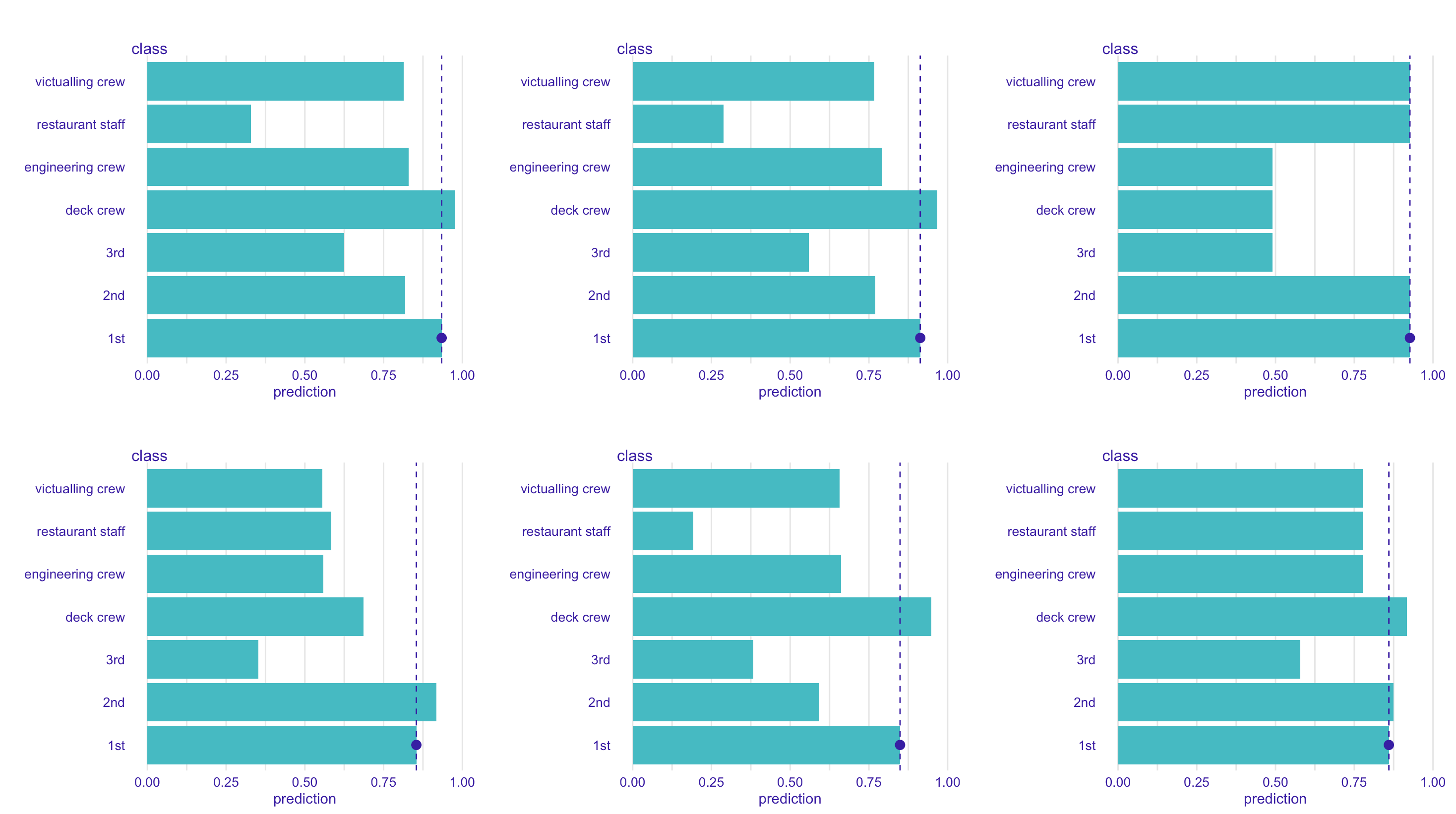• Local Approach : additive (break-point) decomposition

For a standard linear model$$\widehat{m}(\boldsymbol{x}^*) = \widehat{\beta}_0 + \widehat{\boldsymbol{\beta}}^\top\boldsymbol{x}^*=\widehat{\beta}_0+\sum_{j=1}^p \widehat{\beta}_j x_j^*=\overline{y} + \sum_{j=1}^p \underbrace{\widehat{\beta}_j\big(x_j^*-\overline{x}_j\big)}_{=v_j(\boldsymbol{x}^*)}$$
where $$v_j(\boldsymbol{x}^*)$$ will be seen as the  contribution of variable $$j$$ in the prediction, for $$\boldsymbol{x}^*$$.

More generally, Robnik-Šikonja and Kononenk (1997, 2003 and 2008), defined the contribution of the $$j$$-th variable, in $$\boldsymbol{x}^*$$, as$$v_j(\boldsymbol{x}^*)=m(x_1^*,\cdots,,x_{j-1}^*,x^*_j,x_{j+1}^*,\cdots,x^*_p) – \mathbb{E}_{\boldsymbol{X}_{-j}^\perp}[m(x^*_1,\cdots,x^*_{j-1},X_j,x^*_{j+1},\cdots,x^*_p)]$$such that$$m(\boldsymbol{x}^*) = \mathbb{E}_{}\big[m(\boldsymbol{X})\big] + \sum_{j=1}^pv_j(\boldsymbol{x}^*)$$therefore, for a linear model $$\displaystyle{v_j(\boldsymbol{x}^*) = \beta_j\big( x_j^* – \mathbb{E}_{\boldsymbol{X}_{-j}^\perp} [X_j]\big)}$$ and $$\widehat{v}_j(\boldsymbol{x}^*) =\widehat{\beta}_j \big( x^*_j-\overline{x}_j\big)$$.

But more generally$$\displaystyle{v_j(\boldsymbol{x}^*)=m(\boldsymbol{x}^*) – \mathbb{E}_{\boldsymbol{X}_{-j}^\perp}[m(\boldsymbol{x}^*_{-j},X_j))]}$$ where we can write $$m(\boldsymbol{x}^*)$$ as $$\mathbb{E}_{\boldsymbol{X}}[m(\boldsymbol{x}^*)]$$, i.e.
$$v_j(\boldsymbol{x}^*)=\begin{cases}\mathbb{E}_{\boldsymbol{X}}\big[m(\boldsymbol{X})\big\vert x^*_1,\cdots,x^*_p\big] -\mathbb{E}_{\boldsymbol{X}_{-j}^\perp}\big[m(\boldsymbol{X})\big\vert x^*_1,\cdots,x^*_{j-1},x^*_{j+1},\cdots,x^*_p\big]\\\mathbb{E}_{\boldsymbol{X}}\big[m(\boldsymbol{X})\big\vert \boldsymbol{x}^*\big] – \mathbb{E}_{\boldsymbol{X}_{-j}^\perp}\big[m(\boldsymbol{X})\big\vert\boldsymbol{x}^*_{-j}\big]\end{cases}$$

The contribution of the $$j$$-th variable, in $$\boldsymbol{x}^*$$, us$$\gamma_j^{bd}(\boldsymbol{x}^*)=v_j(\boldsymbol{x}^*)=\mathbb{E}_{\boldsymbol{X}}\big[m(\boldsymbol{X})\big\vert \boldsymbol{x}^*\big] -\mathbb{E}_{\boldsymbol{X}_{-j}^\perp}\big[m(\boldsymbol{X})\big\vert\boldsymbol{x}^*_{-j}\big]$$This is clearly a ceteris paribus approach, as we can see below (where I will simply draw a couple of pictures). Consider a set  $$\mathcal{X}$$ such that $$0\leq x_2\leq x_1\leq 1$$. Below, points can be in the blue region, not in the red one,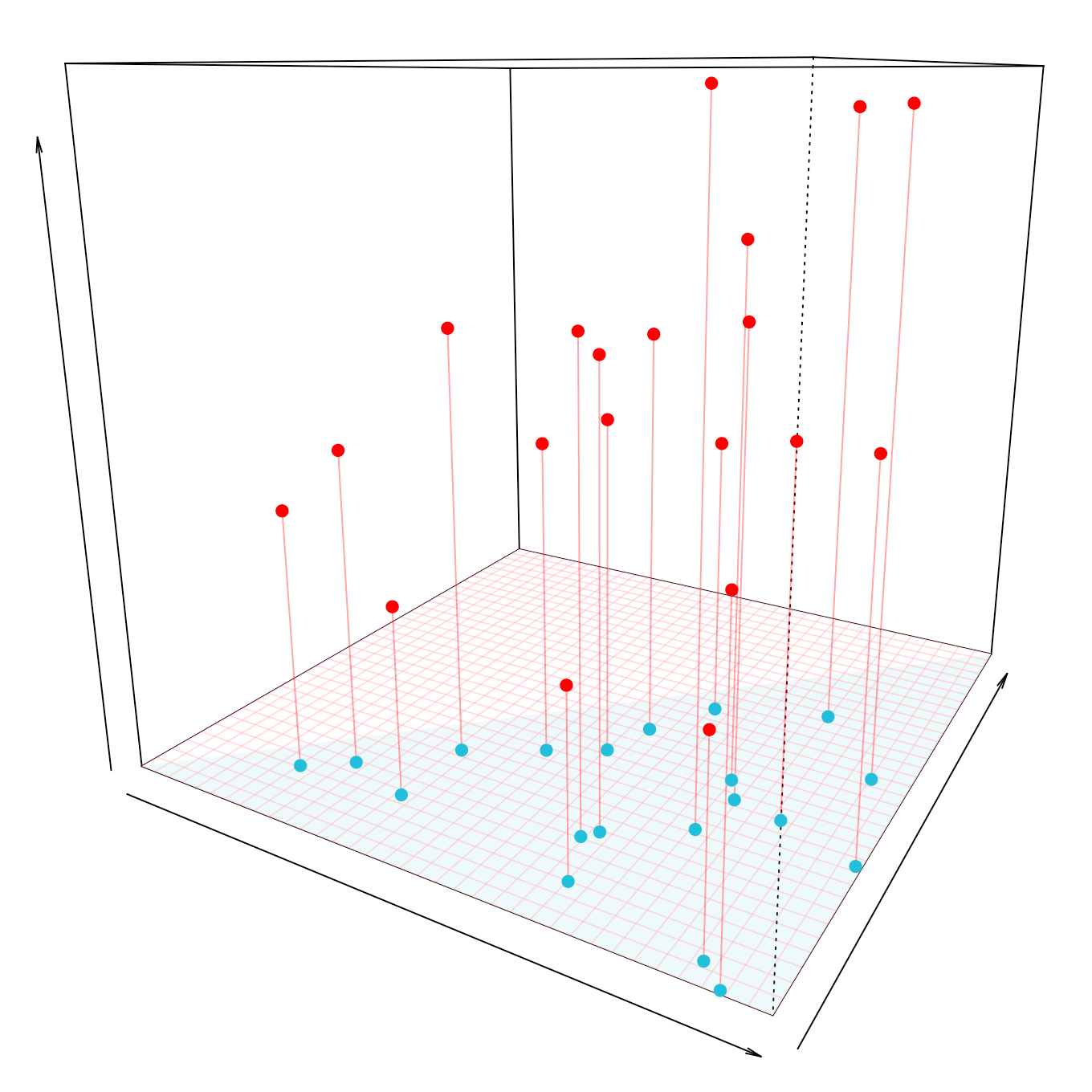We want to understand the prediction given by the least squares regression, for one of the points, i.e. to get a break-down decomposition of $$\widehat{y}$$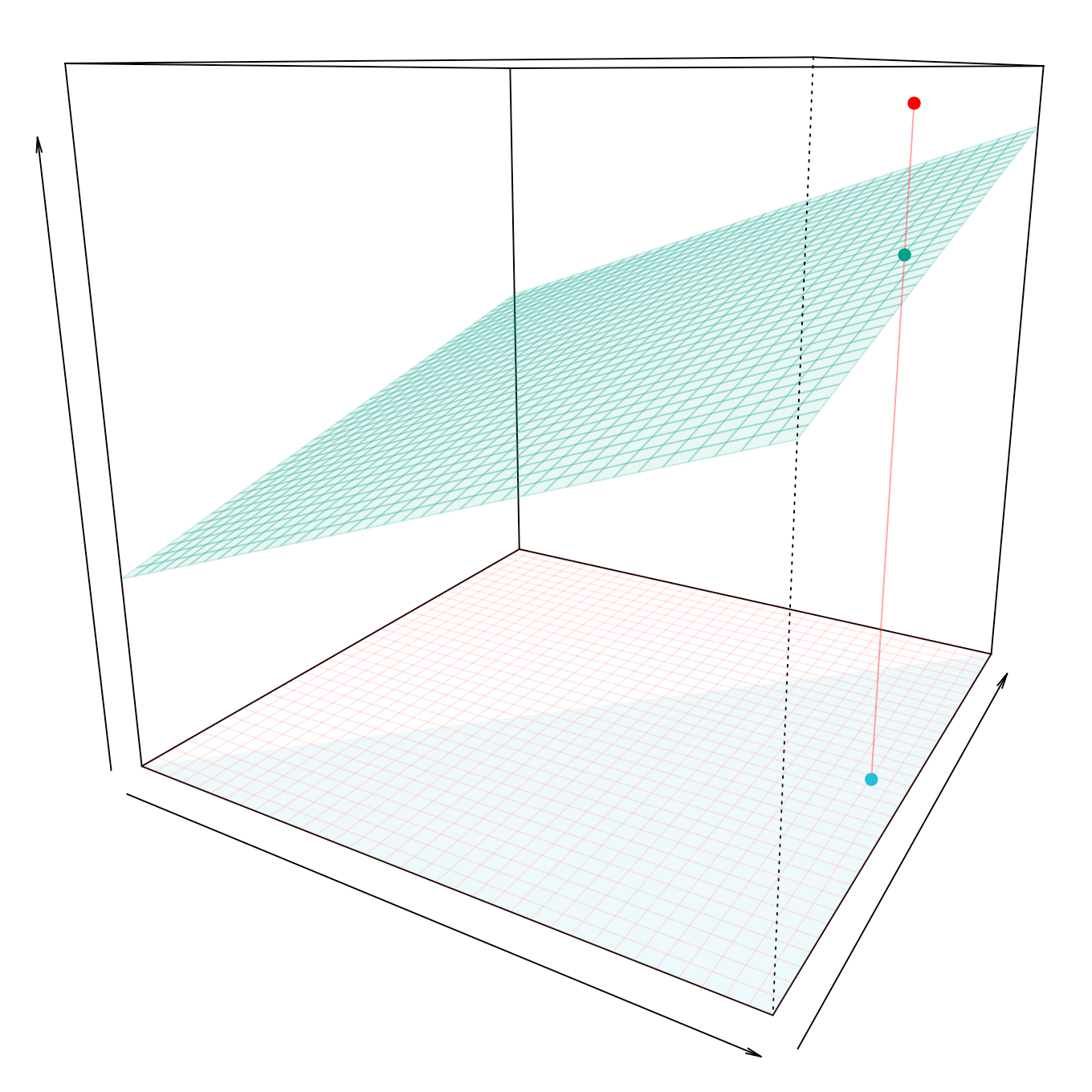First, we compute the average value of $$y$$Then, we will compute averages, ceteris paribus, the first one being $$\displaystyle{ \mathbb{E}_{X_1^\perp}\big[m(x_1^*,X_2)\big] \approx \frac{1}{n}\sum_{i=1}^n m(x_1^*,x_{2,i})}$$where the sum is obtained when $$x_1^*$$ is fixed (why not)and the second one is$$\displaystyle{\mathbb{E}_{X_2^\perp}\big[m(X_1,x_2^*)\big] \approx \frac{1}{n}\sum_{i=1}^n m(x_{1,i},x_2^*)}$$i.e. we sum when $$x_2^*$$ is fixed, which is rather odd in this example since we consider some pseudo-observations $$(x_{1,i},x_2^*)$$ that are clearly in the red area, where we should have no points…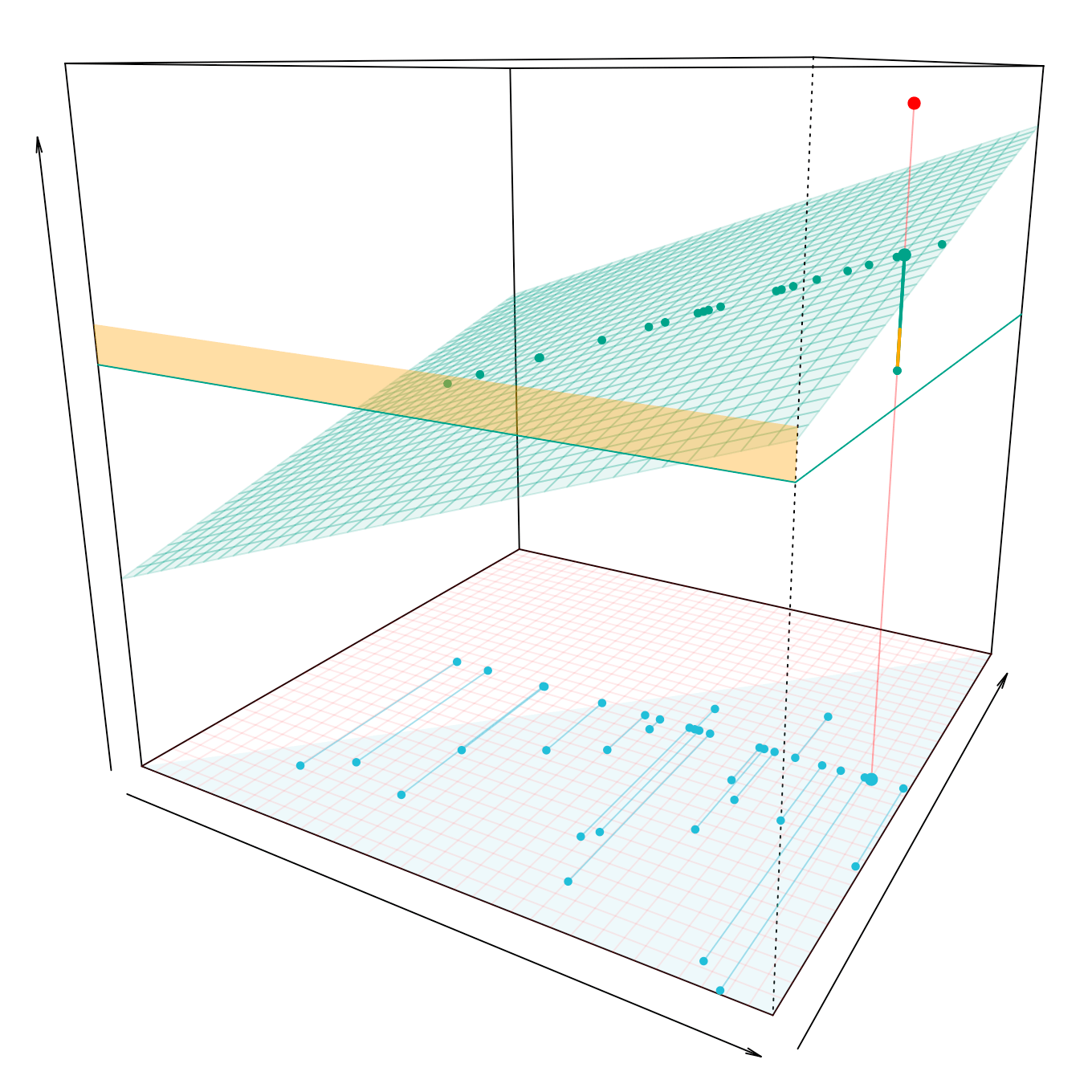In the last two cases, the contribution will be the conditional mean, from which the global mean is subtracted (this is the height that can be seen in yellow). And we can show that the mean, to which we add the two contributions, gives the predicted value.

On the Titanic data, for Kate and just the logistic regression

 bd_glm_kate = DALEX::predict_parts(explainer = titanic_glm_exp,
new_observation = newbase[1,],
type = "break_down",
order = c("gender","class", "age",
"parch", "sibsp", "embarked"))
plot(bd_glm_kate)To go a little further, note that we can define the contribution of the $$j$$-th conditional variable to a group of variables $$S\subset\{1,\cdots,p\}\backslash\{j\}$$, in $$\boldsymbol{x}^*$$, est$$\Delta_{j|S}(\boldsymbol{x}^*)=\begin{cases}\mathbb{E}_{\boldsymbol{X}^\perp_S,X^\perp_j}\big[m(\boldsymbol{X})\big\vert\boldsymbol{x} ^*_S, x^*_j\big] – \mathbb{E}_{\boldsymbol{X}_S^\perp}\big[m(\boldsymbol{X})\big\vert\boldsymbol{x}^*_S\big]\\\mathbb{E}_{\boldsymbol{X}^\perp_{S \cup\{j\}}}\big[ m(\boldsymbol{X})\big\vert \boldsymbol{x}^*_{S\cup\{j\}}\big] -\mathbb{E}_{\boldsymbol{X}_S^\perp}\big[m(\boldsymbol{X})\big\vert\boldsymbol{x}^*_S\big]\end{cases}$$so that $$v_j(\boldsymbol{x}^*)=\Delta_{j|\{1,2,\cdots,p\}\backslash\{j\}}=\Delta_{j|-j}$$.

More generally, define also $$\Delta_{K|S}(\boldsymbol{x}^*)$$, or $$\Delta_{i,j|S}(\boldsymbol{x}^*)$$ (which would allow further analysis of possible interractions, but we’ll pass quickly).

• Approche locale : la décomposition de Shapley

As a reminder, in a (very) general context, $$\forall S\subset\{1,\ldots,p\}$$, we have a $$\text{val}(S)$$ function, and we are looking for $$\phi_j(\text{val})$$ contributions, checking some criteria

• efficiency: $$\displaystyle{\sum_{j=1}^p \phi_j(\text{val}) = \text{val}(\{1,\ldots,p\})}$$
• symmetry: if $$\text{val}\left(S\cup\{j\}\right)=\text{val}\left(S\cup\{k\}\right)$$, $$\forall S\subseteq\{1,\ldots,p\}\setminus\{j,k\}$$, then $$\phi_j=\phi_k$$
• dummy: if $$\text{val}\left(S\cup\{j\}\right)=\text{val}\left(S\right)$$, $$\forall S\subseteq\{1,\ldots,p\}$$, then $$\phi_j=0$$
• additivity: if $$\text{val}^{(1)}$$ and $$\text{val}^{(2)}$$ have the decompositions $$\phi^{(1)}$$ and $$\phi^{(2)}$$, then $$\text{val}^{(1)}+\text{val}^{(2)}$$ has the decomposition $$\phi^{(1)} + [latex]\phi^{(2)}$$

Shapley (1953) proved that the ony functions satisfying those criteria are$$\phi_j(\text{val})=\sum_{S\subseteq\{1,\ldots,p\}\setminus\{j\}}\frac{|S|!\left(p-|S|-1\right)!}{p!}\left(\text{val}\left(S\cup\{j\}\right)-\text{val}(S)\right)$$or encore$$\phi_{j}(\text{val})=\frac{1}{p} \sum_{S \subseteq\{1,\ldots,p\}\setminus\{j\}}\binom{p-1}{|S|}^{-1}(\text{val}(S\cup\{j\})-\text{val}(S))$$

Here, we will use $$\text{val}(S)=\mathbb{E}_{\boldsymbol{X}_S^\perp}\big[m(\boldsymbol{X})\big\vert \boldsymbol{x}^*_S\big]$$

The contribution of the $$j$$-th variable, in $$\boldsymbol{x}^*$$, is therefore$$\gamma_j^{shap}(\boldsymbol{x}^*)=\frac{1}{p} \sum_{S \subseteq\{1,\ldots,p\}\setminus\{j\}}\binom{p-1}{|S|}^{-1} \Delta_{j|S}(\boldsymbol{x}^*)$$

• local accuracy: $$\displaystyle{\sum_{j=1}^p \gamma_j^{shap}(\boldsymbol{x}^*)=m(\boldsymbol{x}^*)-\mathbb{E}\big[m(\boldsymbol{X})\big]}$$
• symmetry: if $$j$$ and $$k$$ are exchangeable, $$\gamma_j^{shap}(\boldsymbol{x}^*)=\gamma_k^{shap}(\boldsymbol{x}^*)$$
\item dummy: if $$X_j$$ does not contribute, $$\gamma_j^{shap}(\boldsymbol{x}^*)=0$$
• additivity: if $$m=m_1+m_2$$, $$\gamma_j^{shap}(\boldsymbol{x}^*;m)=\gamma_j^{shap}(\boldsymbol{x}^*;m_1)+\gamma_j^{shap}(\boldsymbol{x}^*;m_2)$$

Observe that if $$p=2$$, $$\gamma_1^{shap}(\boldsymbol{x}^*)=\Delta_{1|2}(\boldsymbol{x}^*)=\gamma_1^{bd}(\boldsymbol{x}^*)$$

And if $$p\gg2$$, lthe calculations can quickly become heavy. Štrumbelj and Kononenko (2014) have proposed a method using simulations. From $$\boldsymbol{x}^*$$ and an individual $$\boldsymbol{x}_i$$, we construct $$\tilde x_{j}=\begin{cases}x^*_j\text{ with probability }1/2\\x_{i, j}\text{ with probability }1/2\\\end{cases}$$and $$\begin{cases}\boldsymbol{x}^{*+}_i = (\tilde x_{1}, \cdots,x_{j}^*,\cdots,\tilde x_{p})\boldsymbol{x}^{*-}_i = (\tilde x_{1}, \cdots,x_{i,j},\cdots,\tilde x_{p})\end{cases}$$and we note that $$\gamma_j^{shap}(\boldsymbol{x}^*)\approx m(\boldsymbol{x}^{*+}_i )-m(\boldsymbol{x}^{*-}_i)$$, and thus$$\widehat{\gamma}_j^{shap}(\boldsymbol{x}^*) = \frac{1}{s}\sum_{i,\cdots,n\}} m(\boldsymbol{x}^{*+}_i )-m(\boldsymbol{x}^{*-}_i)$$
(we draw at each step an individual $$i$$ in the training dataset, $$s$$ times).

shap_glm = DALEX::predict_parts(explainer = titanic_glm_exp,
new_observation = newbase[1,],
type = "shap",
order = c("gender","class", "age",
"parch", "sibsp", "embarked"))
plot(shap_gLm, show_boxplots=FALSE)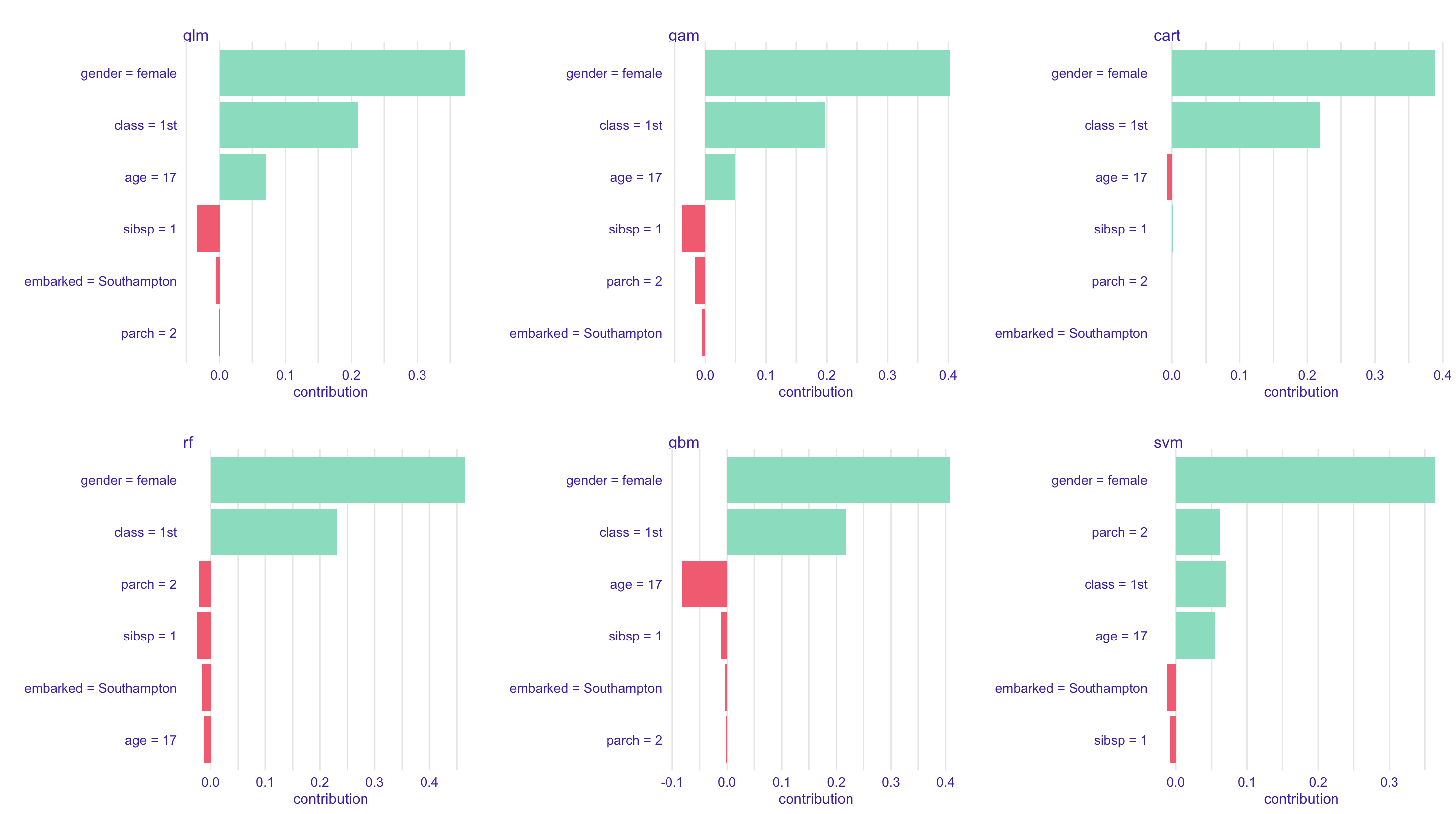or with confidence boxplots

plot(shap_gLm, show_boxplots=TRUE)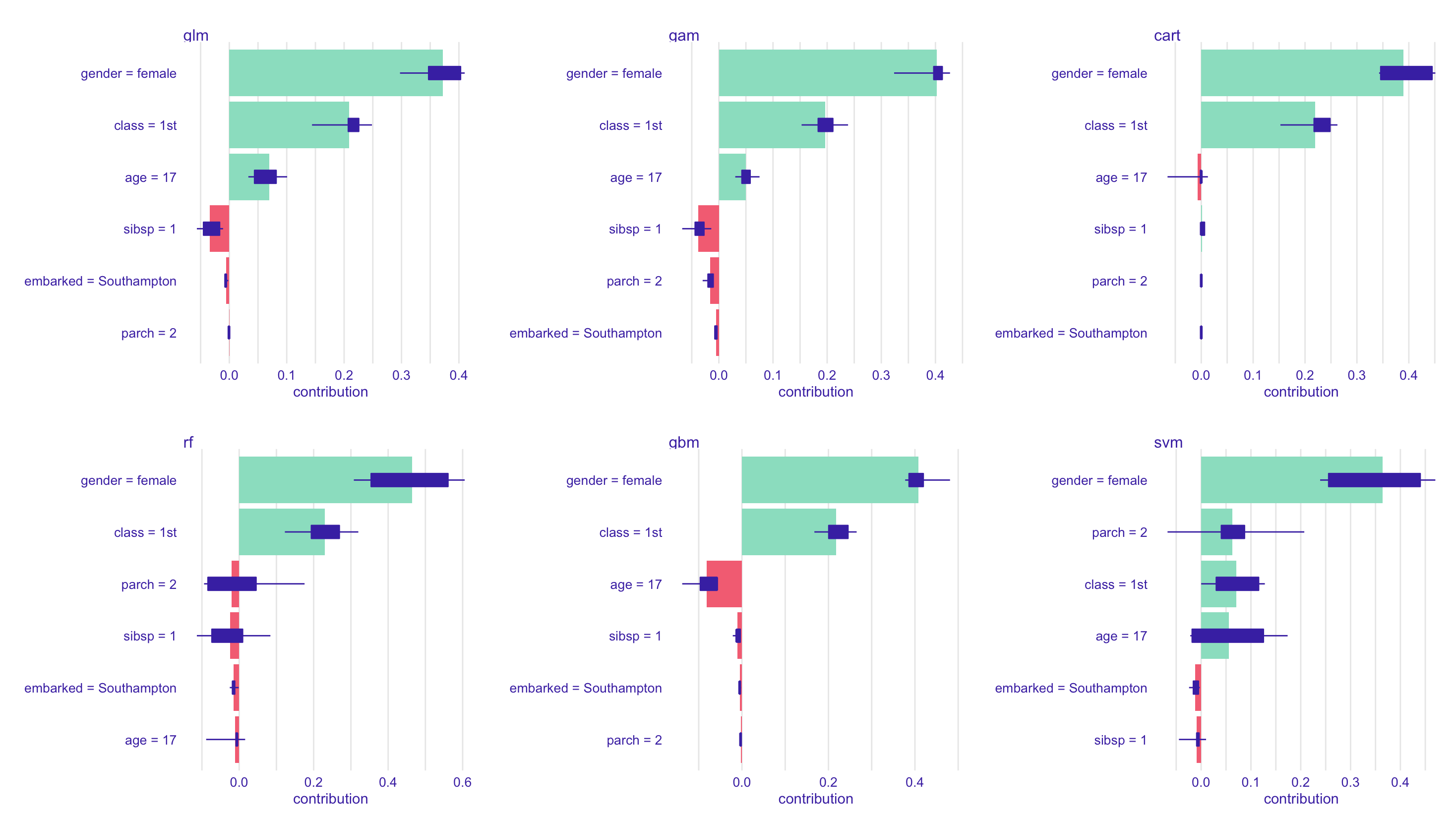Observe that instead of $$\boldsymbol{x}^*$$, we can consider all points in the training setAnd we can actually plot the scatterplot $$\{(x_{i,j},\gamma_j^{shap}(\boldsymbol{x}_i))\}$$ (on the training set) also called “Shapley Dependence Plots”.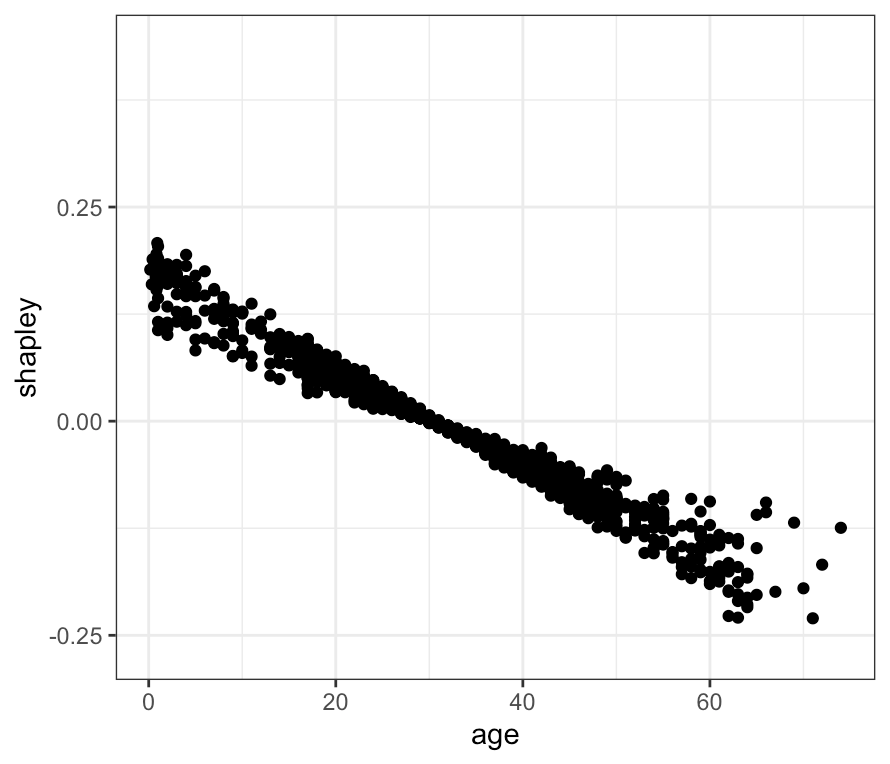or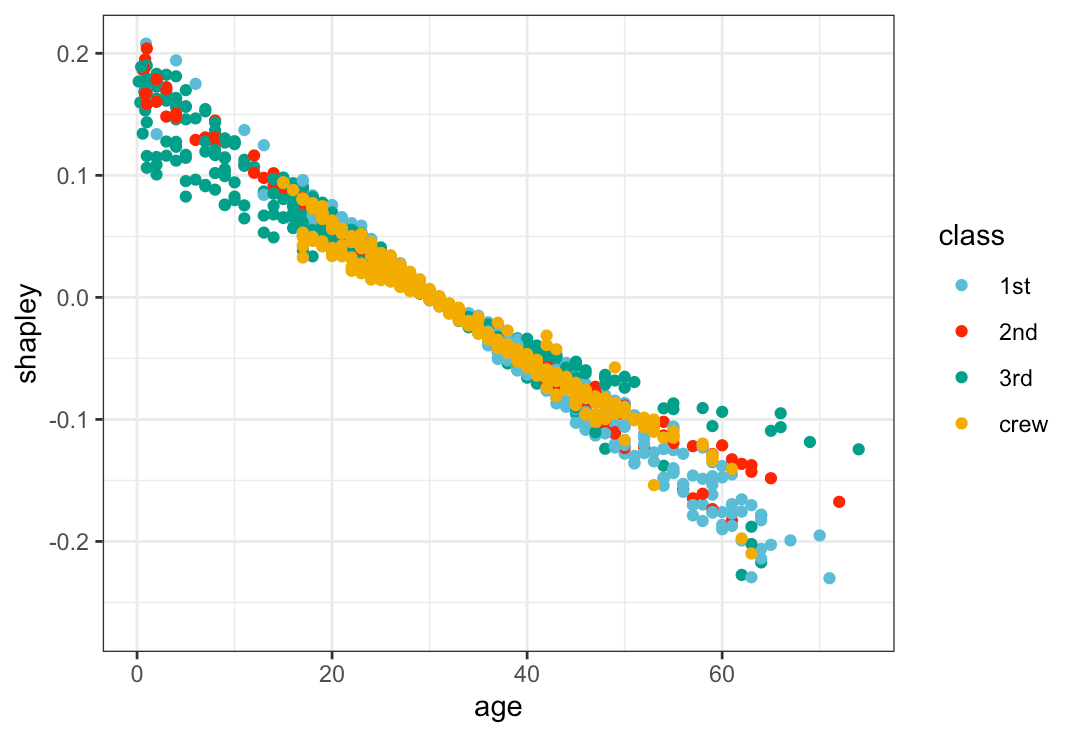Instead of a local vision (at point $$\boldsymbol{x}^*$$) it is actually possible to get a global one..

Štrumbelj and Kononenko (2014), and then Lundberg and Lee (2017) suggested to use the Shapley decomposition to compute a global feature importance function. Shapley Feature Importance is simply$$\gamma_j^{shap} = \frac{1}{n}\sum_{i=1}^n \gamma_j^{shap}(\boldsymbol{x}_i)$$

• Local Approach : LIME (Local Interpretable Model-Agnostic Explanations)

Give a (black box) model $$m$$ defined on $$\mathcal{X}$$, Ribeiro, Singh and Guestrin (2016) suggested to solve$$\underset{m_e\in\mathcal{E}}{\text{argmin}} \big\lbrace\ell_{\boldsymbol{x}^*}(m,m_e)\big\rbrace+\mathcal{P}(m_e)$$where

• $$\mathcal{E}$$ is a subset of models $$\mathcal{X}\to\mathbb{R}$$ that are supposed to be “explainable” (like a linear model, or some tree), or possibly $$\tilde{\mathcal{X}}\to\mathbb{R}$$, where $$\tilde{\mathcal{X}}$$ is a subspace of $$\mathcal{X}$$ (called space for interpretable representation)
• $$\ell_{\boldsymbol{x}^*}$$ is a loss function, defined on the neigborhood of $$\boldsymbol{x}^*$$
• $$\mathcal{P}$$ is a penalty function, increasing in the complexity of the model(I will mention here some old slides used a few years ago to explain models on pictures). Here pictures are our indivudals $$\boldsymbol{x}$$ in dimension $$30000$$ (we have $$100\times100$$ pixels pictures, in coulors, so that the true dimension is three times more. We clearly see on the top right that the first step of the LIME procedure is to simplify our large dimensional individual, by creating a $$6\times$$ object. This approach creates surrogate models (locally)

library(localModel)
library(DALEXtra)
localModel_1_glm = predict_surrogate(explainer = titanic_glm_exp,
new_observation = newbase[1,],
size = 1000,
type = "localModel")
plot_interpretable_feature(localModel_1_glm,"age")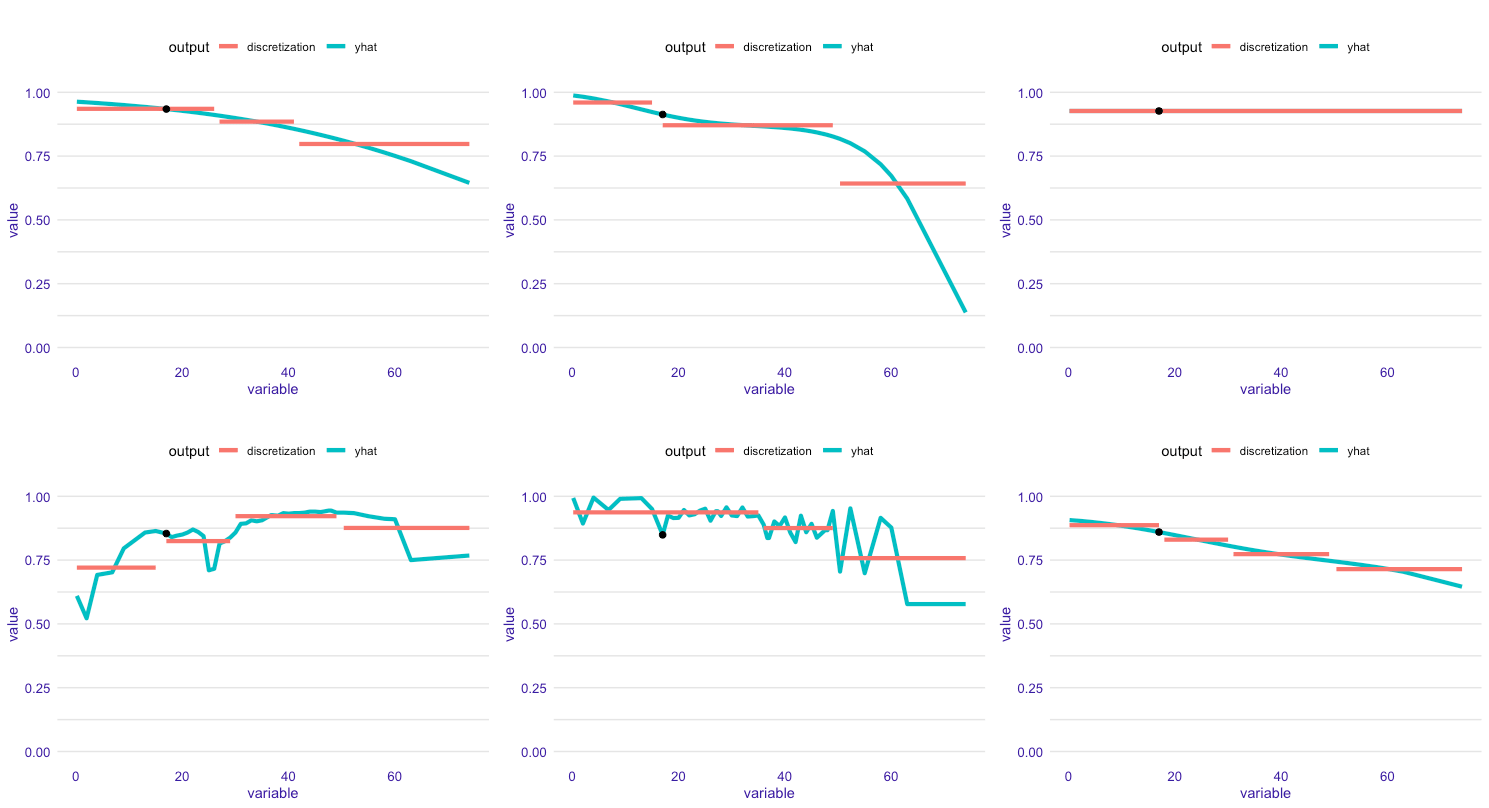• Local Approach : local-diagnostic plots

From our point $$\boldsymbol{x}^*$$, we seek its closest neighbors, and we compare

•  the global distribution of residuals
• the distribution of residuals only for the neighbors of $$\boldsymbol{x}^*$$

using e.g. Gower’s distance $$d_G(\boldsymbol{x}_i,\boldsymbol{x}^*)=\frac{1}{p}\sum_{j=1}^p d_j({x}_{i,j},{x}_j^*),$$where$$d_j({x}_{i,j},{x}_j^*)=\begin{cases}\displaystyle{\frac{|{x}_{i,j}-{x}_j^*|}{\max\{x_j\}-\min\{x_j\}}}, \text{ is }j\text{ continuous}\\\boldsymbol{1}({x}_{i,j}\neq{x}_j^*), \text{ if }j\text{ categorical}\end{cases}$$

res_glm_1 = predict_diagnostics(explainer = titanic_glm_exp,
new_observation = newbase[1,],
neighbors = 25)
plot(res_glm_1)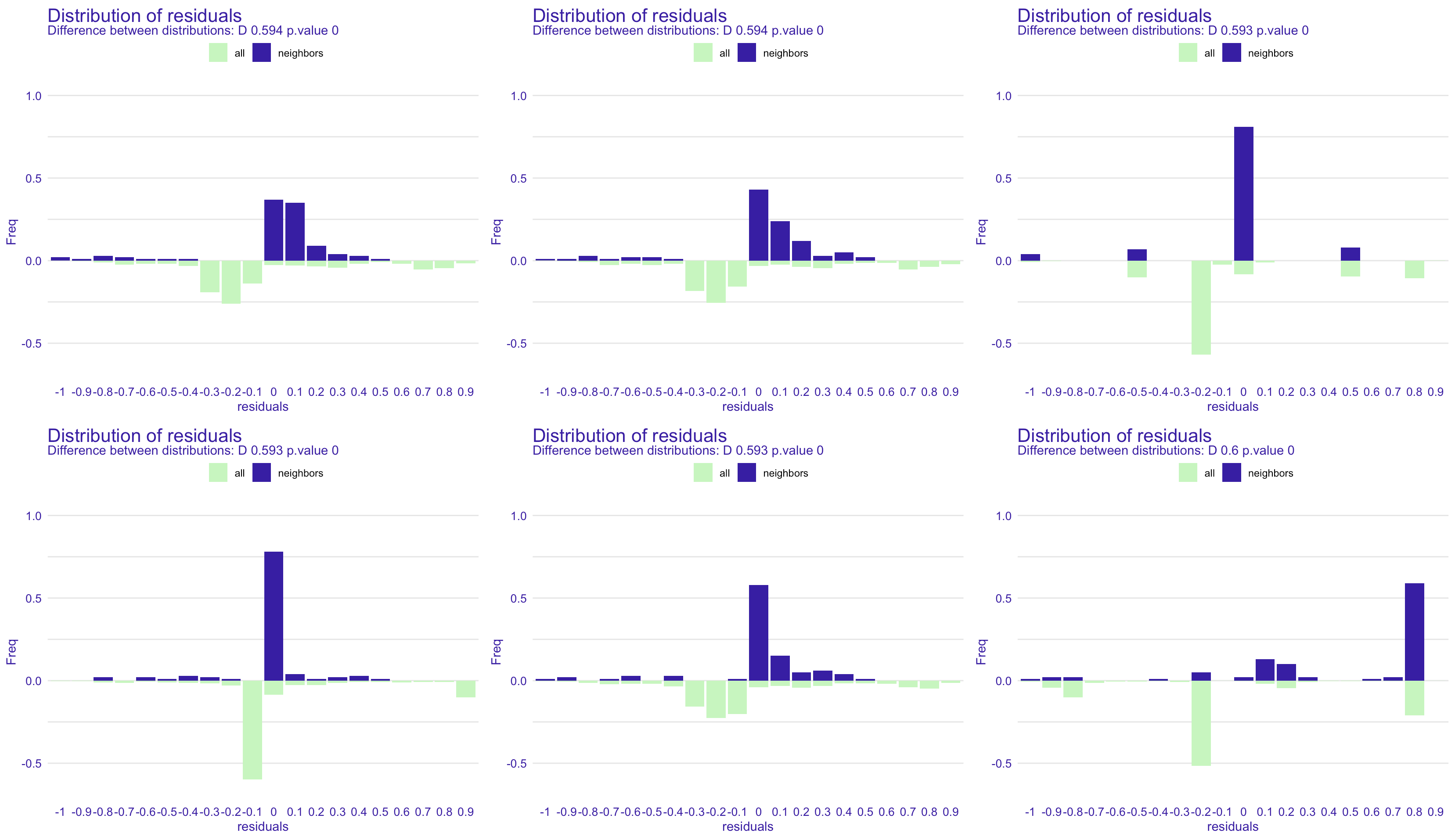with the overall residuals below, in green, and for the neighbors of $$\boldsymbol{x}^*$$ on top, in blue.

We can also plot the ceteris paribus plot of $$\boldsymbol{x}^*$$, e.g. for the age, and the ones of the neighbors (here Kate’s neighbors), with residuals either in red (negative) or green (positive)

res_glm_1_age = predict_diagnostics(explainer = titanic_glm_exp,
new_observation = newbase[1,],
neighbors = 25,
variables = "age")
plot(res_glm_1_age)• Global Approach : Partial dependence plot

The Partial Dependence Plot of variable $$j$$ is function $$\mathcal{X}_j\to\mathbb{R}$$$$p_j(x_j^*)=\mathbb{E}_{X_j^\perp}\big[ m(\boldsymbol{X})|x_j^*\big]$$and its empirical version is$$\widehat{p}_j(x_j^*)=\frac{1}{n} \sum_{i=1}^n {m}(x_j^*,\boldsymbol{x}_{i,-j})=\frac{1}{n}\sum_{i=1}^n \underbrace{{m}_{\boldsymbol{x}_{i}}(x_j^*)}_{\text{ceteris paribus}}$$

pdp_glm = model_profile(explainer = explain_glm,
variables = "age")
plot(pdp_glm)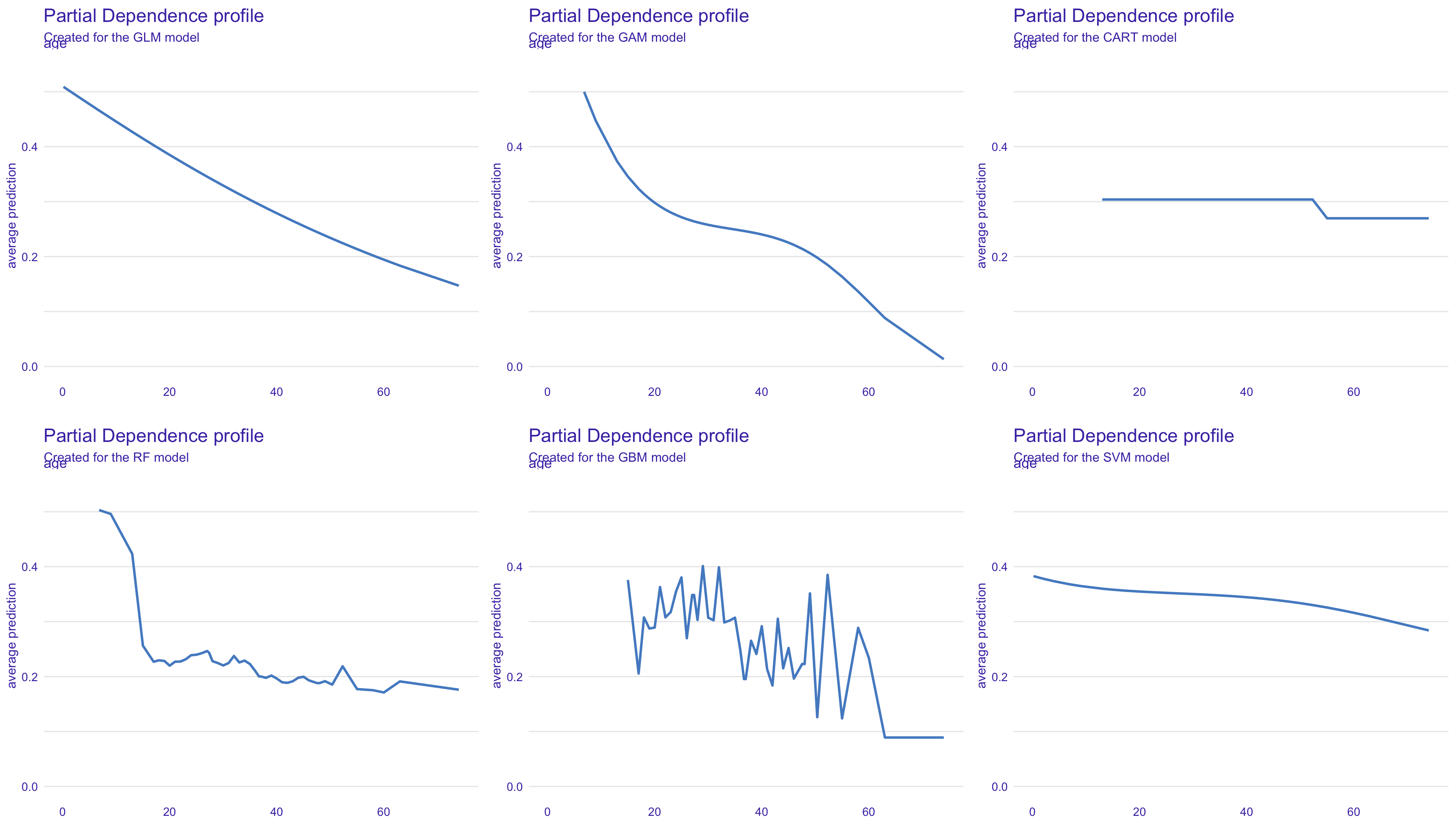where we add a few ceteris paribus curves (the average curve is still the large blue one)Note that instead of the average overall$$\widehat{p}_j(x_j^*)=\frac{1}{n} \sum_{i=1}^n {m}(x_j^*,\boldsymbol{x}_{i,-j})=\frac{1}{n}\sum_{i=1}^n \underbrace{{m}_{\boldsymbol{x}_{i}}(x_j^*)}_{\text{ceteris paribus}}$$it is possible to consider averages on subsets, e.g. for men and women$$\widehat{p}_j(x_j^*)=\frac{1}{n_k}\sum_{i\in\text{group}_k} \underbrace{{m}_{\boldsymbol{x}_{i}}(x_j^*)}_{\text{ceteris paribus}}$$

pdp_glm = model_profile(explainer = explain_glm,
variables = "age", groups = "gender")
plot(pdp_glm)or grouped in two classes in an unsupervised way (the subgroups are here constituted by models, one thus has no coherence between the drawings)

pdp_glm = model_profile(explainer = explain_glm,
variables = "age", k = 3)
plot(pdp_glm)• Global Approach : Local dependence plot

Notice that $$\displaystyle{p_j(x_j^*)=\mathbb{E}_{X_j^\perp}\big[m(\boldsymbol{X})|x_j^*\big]}$$ did not take into account the fact that, generally, $$(\boldsymbol{X}_{-j}|X_j)\overset{\mathcal{L}}{\neq}\boldsymbol{X}_{-j}$$. Therefore, instead of the previous ceteris paribus approach, used to get PDPs, we can consider a mutandis mutatis approach.

Apley and Zhu (2020) defined$${\ell}_j(x_j^*)=\mathbb{E}_{X_j}\big[m(\boldsymbol{X})|x_j^*\big]$$pour l’approche théorique, et la version empirique est alors$$\widehat{{\ell}}_j(x_j^*)=\frac{1}{\text{card}(V(x_j^*))}\sum_{i\in V(x_j^*)} {m}(x_j^*,\boldsymbol{x}_{i,-j})$$où $$V(x_j^*)=\big\lbrace i:d(x_{i,j},x_j^*)\leq \epsilon\big\rbrace$$ est le voisinage, ou$$\widehat{{\ell}}_j(x_j^*)=\frac{1}{\sum_i\omega_i(x_j^*)}\sum_{i=1}^n\omega_i(x_j^*) {m}(x_j^*,\boldsymbol{x}_{i,-j})$$où $$\omega_i(x_j^*)=K_h(x_j^*-x_{i,j})$$ pour une version lissée par noyau.

loc_glm = model_profile(explainer = explain_glm, variables = "age", type="conditional")• Global Approach : Accumulated Local Effects

Define$$a_j(x_j^*)=\int_{-{\infty}}^{x_j^*}\mathbb{E}_{X_j}\left[\frac{\partial m(x_j,\boldsymbol{X}_{-j})}{\partial{x}_j}\Big\vert x_j\right]dx_j$$where here$$\displaystyle{\frac{\partial m(x_j,\boldsymbol{X}_{-j})}{\partial{x}_j}}$$ describes the local change of the model $$m$$ from $$x_j$$ (ceteris paribus), $$m(x_j+dx_j,\boldsymbol{x}_{-j})-m(x_j, \boldsymbol{x}_{-j}) \approx \displaystyle{\frac{\partial m(x_j,\boldsymbol{X}_{-j})}{\partial{x}_{-j}}dx_j$$and we look at the local mean value.

The empirical version is$$\widehat{a}_j(x_j^*)= \alpha+ \sum_{u=1}^{k_j^*}\frac{1}{n_u}\sum_{u:x_{i,j}\in(a_{u-1},a_u]} \left[m(a_k,\boldsymbol{x}_{i,-j})-m(a_{k-1},\boldsymbol{x}_{i,-j})\right]$$(where $$\alpha$$ denotes a normalization constant that insures that $$\mathbb{E}[a_j(X_j)]=0$$).

Apley and Zhu (2020) suggested either a discretization of $$X_j$$ (partition $$\{(a_{k-1},a_k]\}$$), or a smooth version (using a kernel)

acc_glm = model_profile(explainer = explain_glm, variables = "age", type="accumulated")